Main Content

# Detect Cell Using Edge Detection and Morphology

This example shows how to detect a cell using edge detection and basic morphology. An object can be easily detected in an image if the object has sufficient contrast from the background.

### Step 1: Read Image

Read in the `cell.tif` image, which is an image of a prostate cancer cell. Two cells are present in this image, but only one cell can be seen in its entirety. The goal is to detect, or segment, the cell that is completely visible.

```I = imread('cell.tif'); imshow(I) title('Original Image'); text(size(I,2),size(I,1)+15, ... 'Image courtesy of Alan Partin', ... 'FontSize',7,'HorizontalAlignment','right'); text(size(I,2),size(I,1)+25, .... 'Johns Hopkins University', ... 'FontSize',7,'HorizontalAlignment','right');```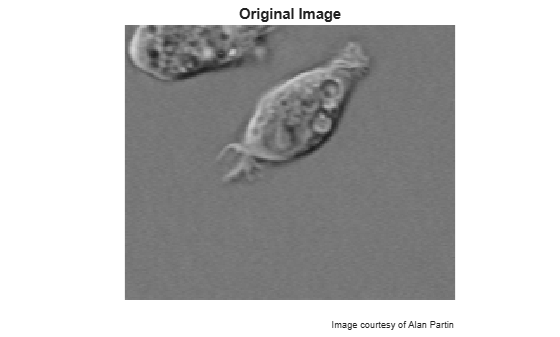### Step 2: Detect Entire Cell

The object to be segmented differs greatly in contrast from the background image. Changes in contrast can be detected by operators that calculate the gradient of an image. To create a binary mask containing the segmented cell, calculate the gradient image and apply a threshold.

Use `edge` and the Sobel operator to calculate the threshold value. Tune the threshold value and use `edge` again to obtain a binary mask that contains the segmented cell.

```[~,threshold] = edge(I,'sobel'); fudgeFactor = 0.5; BWs = edge(I,'sobel',threshold * fudgeFactor);```

Display the resulting binary gradient mask.

```imshow(BWs) title('Binary Gradient Mask')```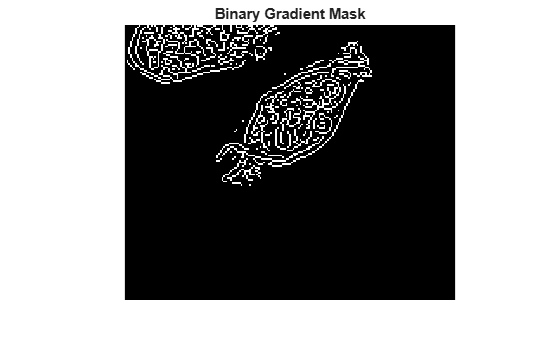### Step 3: Dilate the Image

The binary gradient mask shows lines of high contrast in the image. These lines do not quite delineate the outline of the object of interest. Compared to the original image, there are gaps in the lines surrounding the object in the gradient mask. These linear gaps will disappear if the Sobel image is dilated using linear structuring elements. Create two perpindicular linear structuring elements by using `strel` function.

```se90 = strel('line',3,90); se0 = strel('line',3,0);```

Dilate the binary gradient mask using the vertical structuring element followed by the horizontal structuring element. The `imdilate` function dilates the image.

```BWsdil = imdilate(BWs,[se90 se0]); imshow(BWsdil) title('Dilated Gradient Mask')```### Step 4: Fill Interior Gaps

The dilated gradient mask shows the outline of the cell quite nicely, but there are still holes in the interior of the cell. To fill these holes, use the `imfill` function.

```BWdfill = imfill(BWsdil,'holes'); imshow(BWdfill) title('Binary Image with Filled Holes')```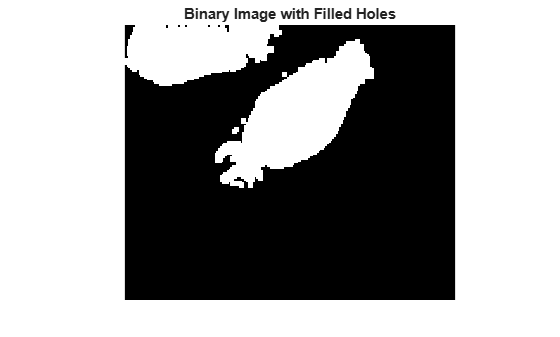### Step 5: Remove Connected Objects on Border

The cell of interest has been successfully segmented, but it is not the only object that has been found. Any objects that are connected to the border of the image can be removed using the `imclearborder` function. To remove diagonal connections, set the connectivity in the `imclearborder` function to `4`.

```BWnobord = imclearborder(BWdfill,4); imshow(BWnobord) title('Cleared Border Image')```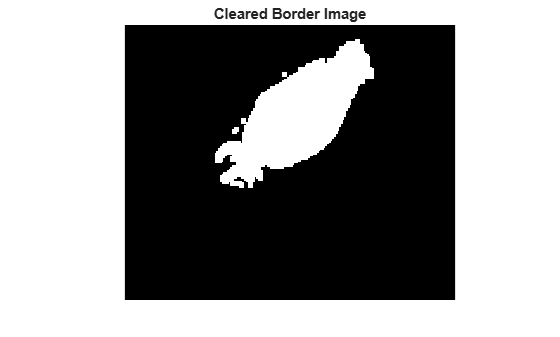### Step 6: Smooth the Object

Finally, in order to make the segmented object look natural, smooth the object by eroding the image twice with a diamond structuring element. Create the diamond structuring element using the `strel` function.

```seD = strel('diamond',1); BWfinal = imerode(BWnobord,seD); BWfinal = imerode(BWfinal,seD); imshow(BWfinal) title('Segmented Image');```### Step 7: Visualize the Segmentation

You can use the `labeloverlay` function to display the mask over the original image.

```imshow(labeloverlay(I,BWfinal)) title('Mask Over Original Image')```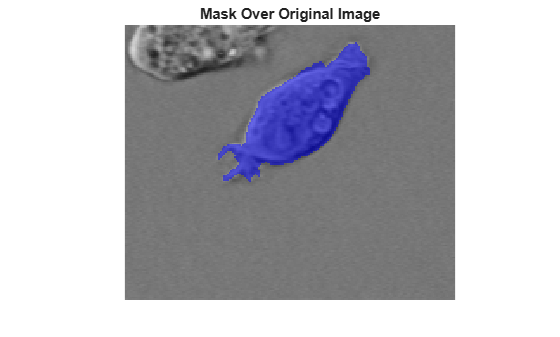An alternate method to display the segmented object is to draw an outline around the segmented cell. Draw an outline by using the `bwperim` function.

```BWoutline = bwperim(BWfinal); Segout = I; Segout(BWoutline) = 255; imshow(Segout) title('Outlined Original Image')```Download ebook